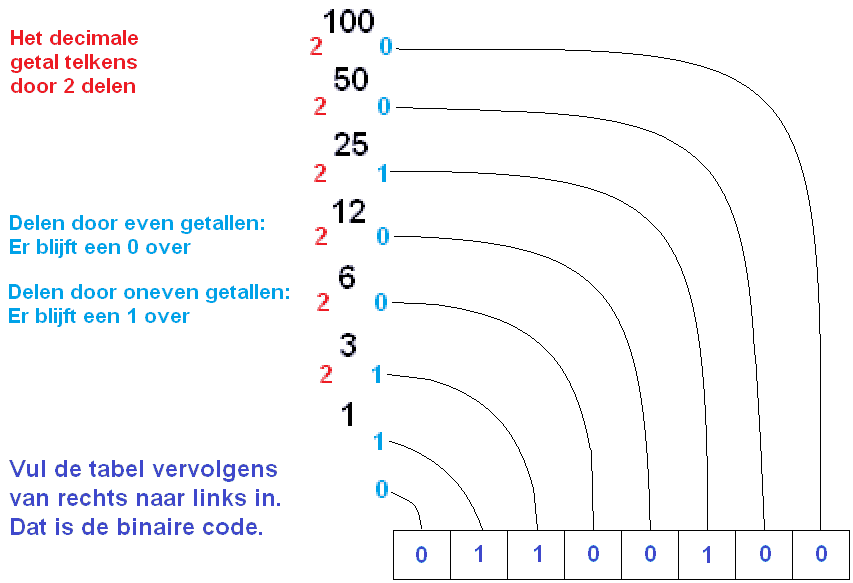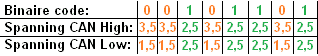Subjects:

• Preface
• Binary
• Decimal
• Convert Binary to Decimal
• Convert Decimal to Binary

Preface:
All computers in the car work with digital electronics: the numbers 1 and 0. When computer A wants to send certain information to computer B via CAN bus, a message of all 1 and 0 is created (binary), then converted to a decimal or hexadecimal message, which is recognized and processed by the computer B. Internally, the processor of the computer always works with ones and zeros. The decimal and hexadecimal codes from the sensors and other computers must therefore always be converted. The 1 means 'on' and a 0 means 'off'.

The binary system is used internally by control devices. Binary codes can also be used for small data transfers, eg switch on (1) or off (0). With larger data transfers from, for example, a temperature sensor, many ones and zeros have to be sent. For example, with a hexadecimal system, the temperature can be passed on much more accurately via the data bus, because many more different temperatures can be passed between 00 and FF than the combinations of ones and zeros in the binary system. The codes can also be converted manually. How this works is explained further on on this page.

Binary:
Binary system: 01
Option: 0 or 1

Practical example: A window switch transmits a decimal code of 252 via the data bus to the control unit. The code is converted by the control unit into a binary code of 11111100. The control unit recognizes that this is the code to open the right front window. The control unit supplies power to the window motor until the switch transmits the code 00000000, or until the window has reached its end position.

 Lv window open: 11111110 Close: 01111111 Open rv window: 11111100 Close: 00111111 Open the window: 11111000 Close: 00011111 Ra window open: 11110000 Close: 00001111 Switches rest position: 00000000 Storage: 11111111

Decimal:
10-digit system: 0123456789
Ability: Between 0 and 255

A decimal number can be seen as a compact binary number. A binary value of: 01100100 is decimal: 100.

16-digit system: 0123456789ABCDEF
Possibility: Between 00 and FF

The hexadecimal system is more extensive than the decimal system. The hexa goes from 0 to 15, while the deci goes from 0 to 9.
The numbers higher than 9 are indicated by letters:
10 = A
11 = OVER
12=C
13 = EASY
14 = E
15=F
This gives more possibilities than the decimal system, and will be ideal for control devices with a lot of data transfer.

Convert Binary to Decimal:To convert decimal to binary: